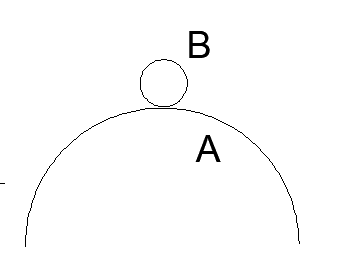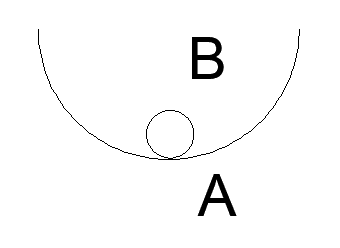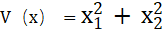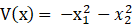• 5.1 李雅普诺夫稳定性的定义 5.2 李雅普诺夫稳定性的基本定理 5.3 线性系统的稳定性分析 5.4 非线性系统的稳定性分析 5.5 Matlab问题
• 详细介绍李雅普诺夫稳定性判据原理，精简易懂。以及如何构建李雅普诺夫函数
• 李雅普诺夫稳定性的基本定理李雅普诺夫稳定性的基本定理李雅普诺夫稳定性的基本定理
• 百度百科-李雅普诺夫稳定性 李雅普诺夫（第二方法）稳定性分析+例题 这俩都很不错

百度百科-李雅普诺夫稳定性

李雅普诺夫（第二方法）稳定性分析+例题     这俩都很不错


展开全文• 分享一个不错的关于李雅普诺夫稳定性分析的资料(入门级) https://wenku.baidu.com/view/82807682b04e852458fb770bf78a6529647d35f9.html
分享一个不错的关于李雅普诺夫稳定性分析的资料(入门级)

https://wenku.baidu.com/view/82807682b04e852458fb770bf78a6529647d35f9.html

展开全文• ## 李雅普诺夫稳定性理论的理解

千次阅读 多人点赞 2019-08-23 10:30:30
最近在学习李雅普诺夫稳定性定理，于是顺便做了一下梳理。
   由于李雅普诺夫第一方法需要求解才能判断系统的稳定性，而大多数情况下，这个解是很难求出来的，所以便有了李雅普诺夫第二法（直接法）。
首先举个例子来说明直接法的基本思想。下图中小球B出在各曲面不同位置时收到微小扰动后的两种运动趋势。
（a）小球受扰后会离开B点，而不会返回B点
（b）小球受扰后，作等幅周期震荡（曲面无摩擦），或作衰减震荡（曲面有摩擦）。（1）小球在（a）中平衡点很容易失去，小球不再回来，因此称该平衡点是不稳定的，该力学系统的稳定性是不好的；而小球在（b）中的平衡点却不会失去，小球作衰减振荡，最终会停在原来的平衡状态，因此称该平衡点是稳定的，该力学系统的稳定性是好的。
（2）受到微小扰动后，小球两种运动趋势不同，（a）中小球离开平衡点，动能是增加的，而（b）中小球离开平衡点，小球作衰减振荡，动能是不断减少的，最终动能会变为零。
以上可总结为：从实际系统的运动可以看出，一个系统如果具有一定的初始能量，若这个系统的能量随时间推移而不断衰减，那么系统迟早会运动到平衡状态。
但实际上寻求系统的能量函数是非常困难的。因此李雅普诺夫提出了一个相似于能量的标量函数，可以理解为“广义能量”
V（x，t）
它是一个标量函数，考虑到能量总大于零，故V(x,t)为一个正定函数。其能量衰减特性用V̇（x，t）表征。利用V及V̇的符号特征，直接对平衡状态稳定性作出判断，无需求出动态方程的解。
对于标量函数的正定性是指V（x）在域S中对所有非零状态有V（x)  > 0，且V（0）=0，则称V（x）在S域内正定，例如负定性即指V（x）在域S中对所有非零状态有V（x）<0,且V（0）=0，则称V（x）在S域内负定。例如以上就是在学习李雅普诺夫定理时做的一点点总结，如有不完善之处还请大家不吝赐教。（ps：写完之后最大感受就是csdn的编辑器写公式也太麻烦了吧）


展开全文• 通过将李雅普诺夫稳定性理论有关定理进行改进，得到判断非线性系统的中心或焦点及估计非自治系统的吸引域的简便而有效的方法．
• 非线性系统的李雅普诺夫稳定性分析,主要是ppt，大家参考消息
• ## 李雅普诺夫稳定性理论

万次阅读 多人点赞 2016-11-10 19:23:13
1892年，俄国学者李雅普诺夫提出的稳定性定理采用了状态向量来描述，适用于单变量，线性，非线性，定常，时变，多变量等系统。目前，李雅普诺夫理论是证明非线性系统稳定性的重要理论依据，也是设计控制算法的重要...
1892年，俄国学者李雅普诺夫提出的稳定性定理采用了状态向量来描述，适用于单变量，线性，非线性，定常，时变，多变量等系统。

目前，李雅普诺夫理论是证明非线性系统稳定性的重要理论依据，也是设计控制算法的重要方法之一。

这里介绍李雅普诺夫直接法（第二法）

基础知识

1、二次型定义及其表达式

形如 f(x,y)=ax2+2bxy+cy2 f(x,y)= ax^2 + 2bxy + cy^2 ，每项的次数都是2。矩阵表示为：
V(x1,x2,⋯,xn)=[x1,x2,⋯,xn]⎡⎣⎢⎢⎢⎢p11,p21,⋯,pn1,p12,p22,⋯,pn2,…,…,⋯,…,p1np2n⋯pnn⎤⎦⎥⎥⎥⎥⎡⎣⎢⎢⎢⎢x1x2⋮xn⎤⎦⎥⎥⎥⎥=xTPxV(x_1,x_2, \cdots  , x_n)=[x_1,x_2,\cdots,x_n] \begin{bmatrix} p_{11},&p_{12},& \dots, &p_{1n} \\   p_{21},& p_{22},& \dots, & p_{2n} \\    \cdots ,& \cdots, & \cdots, & \cdots  \\  p_{n1},&p_{n2},& \dots, &p_{nn}     \end{bmatrix}  \begin{bmatrix}   x_1 \\ x_2 \\ \vdots \\ x_n \end{bmatrix} = x^T P x
其中，P$P$ 为对称矩阵。

2、（二次型）V(x) 判据

（1）、 V(x)$V(x)$正定的充分必要条件是矩阵 P$P$的所有主子式行列式为正。
（2）、若  P$P$ 是奇异矩阵，且它的所有主子式行列式均非负，则  V(x)$V(x)$ 为半正定的。
（3）、如果矩阵 P$P$的奇数阶主子行列式为负值，偶数阶主子行列式为正值，则其为负定的。
（4）、若  P$P$ 正定，则对于任意  x≠0$x \neq 0$,  总有 V(x)>0$V(x) > 0$。

3、任意 V(x) 判据

（1）、则对于任意  x≠0$x \neq 0$,  总有 Q(x)=xTQx>0$Q(x) =x^T Q x> 0$ ，则称 Q(x)$Q(x)$ 为正定函数。
（2）、则对于任意  x$x$,  总有 Q(x)=xTQx≥0$Q(x) =x^T Q x \geq 0$ ， 且存在 x≠0$x \neq 0$ ，使  Q(x)=0$Q(x) =0$，则称 Q(x)$Q(x)$ 为半 正定函数。
（3）、若 −Q(x)$-Q(x)$ 为（半）正定函数，则 Q(x)$Q(x)$ 为（半）负定函数

稳定性定理

一、稳定性定义

1、对系统 x˙=f(x,t)$\dot x = f(x,t)$,若任意给定一个实数 ϵ>0$\epsilon > 0$，总存在另一个实数δ(ϵ,t0)>0$\delta (\epsilon,t_0)>0$ ，使当 初始条件 ||x（t0)||<δ$|| x（t_0) || < \delta$ 时，系统的状态 ||x（t）||<δ，∀t≥to$||x（t）||< \delta ，\forall t \geq t_o$  , 则称系统的平衡状态xs$x_s$  是稳定的。否则，就是不稳定的。
2、一致稳定性
如果系统的平衡状态是稳定的，且 δ$\delta$  与t0$t_0$ 无关，（若任意给定一个实数 ϵ>0$\epsilon > 0$，总存在另一个实数δ(ϵ)>0$\delta (\epsilon)>0$ ，使当 初始条件 ||x（t0)||<δ$|| x（t_0) || < \delta$ 时，系统的状态 ||x（t）||<δ，∀t≥to$||x（t）||< \delta ，\forall t \geq t_o$ ），则该平衡状态是一致稳定的。
若定常系统的平衡状态是稳定的，则一定是一致稳定的。

3、渐近稳定性
若 xe$x_e$是系统的一个稳定点，对任意 t0$t_0$，存在正常数
δ(t0)∈R+$\delta(t_0) \in R^+$，当 x(t0)<δ$x(t_0) < \delta$， 系统的状态收敛于0，即 limt→∞||x−xe||=0  \lim\limits_{t\rightarrow{\infty}} ||x-x_e||=0
则系统是渐进稳定的。

4、指数稳定性
对一个系统而言，如果存在正常数 α,λ∈R+$\alpha,\lambda \in R^+$，当初始位置在以原点为中心的球域范围内，即 x(t0)∈Br(o,r)$x(t_0) \in B_r(o,r)$ 时，系统的状态x(t)$x(t)$具有以下的包络线:
||x(t)||≤||x(t0)||e−λ(t−t0)||x(t)|| \leq ||x(t_0)|| e^{- \lambda (t-t_0)} ,则称平衡点 xs=0$x_s =0$  是指数稳定的，其中正常数  λ$\lambda$ 称为指数收敛率。

二、稳定性定理

李雅普诺夫第二法是从能量的观点出发得来的。任何物理系统的运动都要消耗能量，并且能量总是大于零的。对于一个不受外部作用的系统，如果系统的能量，随系统的运动和时间的增长而连续地减小，一直到平衡状态为止，则系统的能量将减少到最小，那么这个系统是渐近稳定的。

1、局部稳定性定理
设x=0$x=0$是系统的平衡点，如果对于球域 BR$B_R$，存在一个标量函数 V(x)$V(x)$ ，它具有一阶连续偏导数，且满足：
（1）、函数V(x)$V(x)$在球域BR$B_R$上是正定的
（2）、若函数 V(x)$V(x)$关于时间的导数在球域BR$B_R$ 上是半负定的，则平衡点x=0$x=0$是局部稳定的
（3）、若函数V(x)$V(x)$关于时间的导数在球域 BR$B_R$上是负定的，则平衡点 x=0$x=0$是局部渐近稳定的

2、全局稳定性定理

设x=0$x=0$是系统的平衡点，存在一个标量函数 V(x)$V(x)$ ，它具有一阶连续偏导数，且满足：
（1）、函数V(x)$V(x)$ 是正定的
（2）、 V(x) 正则，即当 ||x||→∞$||x|| \rightarrow \infty$ 时，  V(x)→∞$V(x) \rightarrow \infty$  。
（3）、若函数 V(x)$V(x)$关于时间的导数是半负定的，则平衡点x=0$x=0$是全局稳定的
（4）、若函数V(x)$V(x)$关于时间的导数是负定的，则平衡点 x=0$x=0$是全局渐近稳定的

3、全局指数稳定性定理
设x=0$x=0$是系统的平衡点，存在一个标量函数 V(x)$V(x)$ ，它具有一阶连续偏导数，且满足：
（1）、函数V(x)$V(x)$ 是正定的
（2）、 V(x) 正则，即当 ||x||→∞$||x|| \rightarrow \infty$ 时，  V(x)→∞$V(x) \rightarrow \infty$  。
（3）、若函数 V(x)$V(x)$关于时间的导数是半负定的
（4）、存在两个正数λ1$\lambda_1$ 和λ2$\lambda_2$  ，分别使得
V(x)≤λ1||x||2,V˙(x)≤λ2||x||2V(x) \leq \lambda_1 ||x||^2, \dot V(x) \leq \lambda_2 ||x||^2
则平衡点 x=0$x=0$是全局指数稳定的，指数收敛率为λ1λ2$\frac {\lambda_1}{\lambda_2}$ 
展开全文非线性系统 控制方法
• 飞机的稳定性是飞行动力学的重要组成部分，基于飞机空气动力和动力学方程的非线性，将李雅普诺夫稳定性分析方法应用于飞机在定常大迎角飞行状态的稳定性分析，该方法克服了小迎角的局限性，在某型号设计中得到了具体...
• 多智能体系统中基于李雅普诺夫稳定性的协同容错控制，周卓夫，黄志武，协同一致性问题为分布式多智能体系统的基础问题，网络化多智能体系统可通过协同控制律实现状态一致。单个智能体的执行器故障可通
• 一个控制系统就和一个社会一样，稳定性是首先要解决的重要问题，是其他一切工作的基础。稳定性问题的字面意思很好理解了，那就是系统在受到扰动后，能否能有能力在平衡态继续工作。大家都知道，历史上社会改革成本很...现代控制理论
• 哈尔滨工程大学自动控制原理，第5章李雅普诺夫稳定性分析
• 讨论了矢量微分方程 =?（t，x）的零解的稳定性，对李雅普诺夫函数V（t，x）的限制条件作了改进，不再要求 负定，但对V（t，x）的要求也有所改变，推广了扰动微分方程组零解稳定性的若干判定定理。
• 俄国学者李雅普诺夫提出的稳定性定理采用了状态向量来描述，适用于单变量，线性，非线性，定常，时变，多变量等系统，该方法称为李雅普诺夫法，有两种分类： （1）李氏第一法（间接法）：求解特征方程的特征值； ...
• 的两个圆，如果初始状态都在小圆内（D点和E点），李雅普诺夫下的稳定性是指最终稳定在大圆之内，渐进稳定性是指最终会回到原点。 LTI系统 之前提到的可以通过判断 x ˙ = A x \dot{x} = Ax x ˙ = A x 中的A...
• 李雅普诺夫七类稳定性的示意图.pptx，可以形象的讲解李雅普诺夫稳定性的定义，并配有示意图，包括一致渐进大范围等
• 基于田玉平等《自动控制原理》，依据学习经验重述，内容：1.背景和思路 2.李雅普诺夫第二方法 3.李雅普诺夫稳定性分析 4.例题
• PAGE / NUMPAGES 基于MATLAB的李雅普诺夫第二法稳定性分析 引言对于一个给定的控制系统稳定性是系统的一个重要特性稳定性是系统正常工作的前提是系统的一个动态属性在控制理论工程中无论是调节器理论观测器理论还是...
• 经过解密后的李雅普诺夫文档，比较详细地介绍了李雅普诺夫稳定性Lyapunov
• 线性时不变系统稳定性分析李雅普诺夫直接法的计算机判定.caj 稳定性理论，自动化，仿真，毕业设计参考文献，中国知网收费文献，免费送给您！！电气自动化，工科控制专用！！！
• https://www.jianshu.com/p/942e2ddc5a16
• 常微分方程定性与稳定性理论，控制专业研究生可以参考一下，里面有李雅普诺夫稳定性的详细介绍
• 教会电力系统稳定性的研究和说明，能够清楚名曲的知道电力系统的状态
• 李雅普诺夫稳定性理论 1.前期铺垫~ ！：稳定性是系统正常工作的重要特性 稳定性，是描述初始条件（不一定为0）下，系统是否具有收敛性，与输入作用无关 在经典控制理论中，有代数判据（劳斯、霍尔维茨）、奈奎斯特......